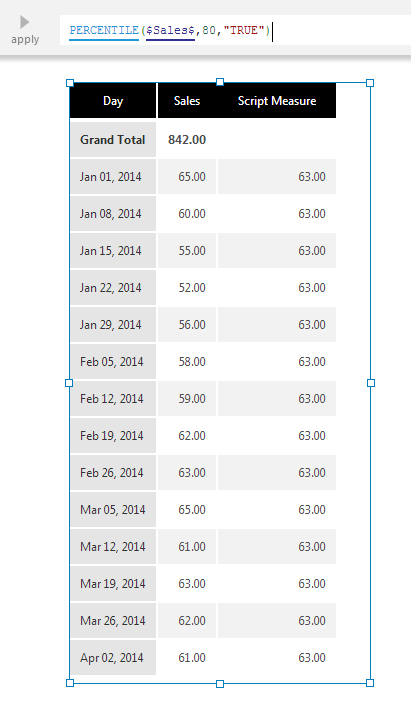# Percentile

Contents[Hide]

The PERCENTILE function returns a scalar value below which the specified percentage of input data values fall. The closest input value that satisfies the condition is returned.Percentile

## 1. Syntax

```PERCENTILE(d0,s0,s1,Alignments...)
```

## 2. Input

The Percentile function requires the following input:

• d0 - The set of data values for which the Percentile is calculated.

## 3. Parameters

The Percentile function requires the following parameters:

• s0 - The percentage value used in Percentile calculations. Default value is 80.
• s1 - Indicates whether to include the two extreme percent values in the calculation. Default value is True.
• Alignments... (One or More, Optional) – Hierarchy placeholders to be used as the alignment axis.

## 4. Output

The Percentile formula generates the following output:

• Percentile - A single value representing the specified percentile.

## 5. See also

Dundas Data Visualization, Inc.
500-250 Ferrand Drive
Toronto, ON, Canada
M3C 3G8

North America: 1.800.463.1492
International: 1.416.467.5100

Dundas Support Hours: 7am-6pm, ET, Mon-Fri